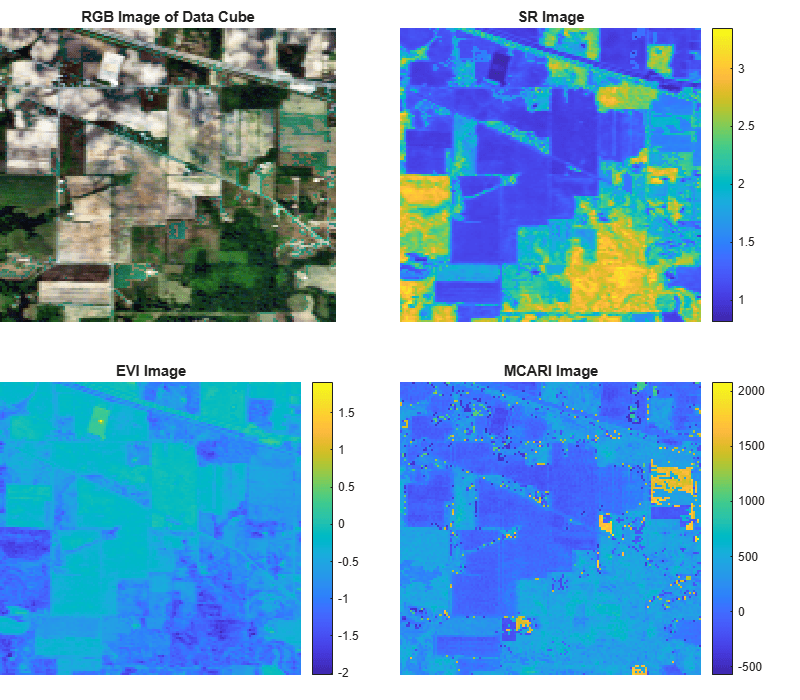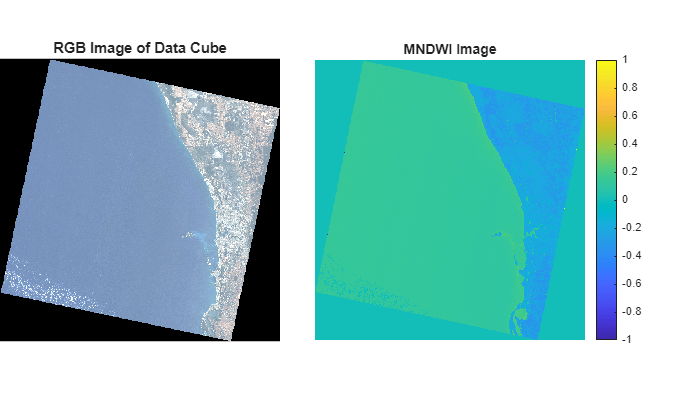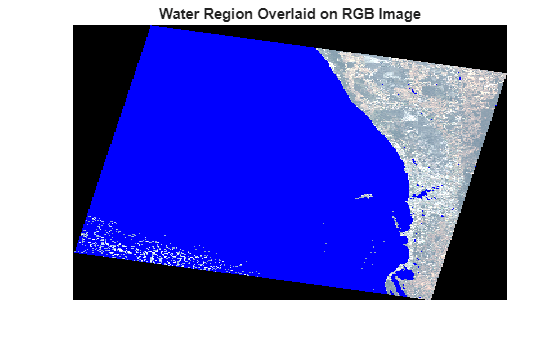# spectralIndices

Compute hyperspectral indices

## Syntax

``indices = spectralIndices(hcube)``
``indices = spectralIndices(hcube,indexNames)``
``indices = spectralIndices(hcube,'all')``
``indices = spectralIndices(___,'BlockSize',blocksize)``

## Description

example

````indices = spectralIndices(hcube)` computes the greenness indices: enhanced vegetation index (EVI), modified chlorophyll absorption ratio index (MCARI), and simple ratio (SR) index of a hyperspectral data. The function reads the data cube and the wavelength values stored in the hypercube object `hcube` to compute the greenness indices.```

example

````indices = spectralIndices(hcube,indexNames)` computes one or more spectral indices specified by `indexNames`.```
````indices = spectralIndices(hcube,'all')` computes all the supported spectral indices.```
````indices = spectralIndices(___,'BlockSize',blocksize)` specifies the block size for block processing of the hyperspectral data cube by using the name-value pair argument `'BlockSize'`. You can specify the `'BlockSize'` name-value pair argument in addition to the input arguments in the previous syntaxes.The function divides the input image into distinct blocks, processes each block, and then concatenates the processed output of each block to form the output matrix. Hyperspectral images are multi-dimensional data sets that can be too large to fit in system memory in their entirety. This can cause the system to run out of memory while running the `spectralIndices` function. If you encounter such an issue, perform block processing by using this syntax.For example, `spectralIndices(hcube,'BlockSize',[50 50])` divides the input image into non-overlapping blocks of size 50-by-50 and then computes the spectral indices for pixels in each block. NoteTo perform block processing by specifying the `'BlockSize'` name-value pair argument, you must have MATLAB® R2021a or a later release. NoteThis function requires the Image Processing Toolbox™ Hyperspectral Imaging Library. You can install the Image Processing Toolbox Hyperspectral Imaging Library from Add-On Explorer. For more information about installing add-ons, see Get and Manage Add-Ons. ```

## Examples

collapse all

Read hyperspectral data into the workspace.

`hcube = hypercube('indian_pines.dat');`

Compute the spectral indices value for each pixel in the data cube. By default, the `spectralIndices` function returns the simple ratio (SR) index, enhanced vegetation index (EVI), and modified chlorophyll absorption ratio index (MCARI).

`indices = spectralIndices(hcube);`

Inspect the index names in the output struct `indices`. Read the corresponding index images returned at the output.

`indices.IndexName`
```ans = "Simple Ratio (SR)" ```
```ans = "Enhanced Vegetation Index (EVI)" ```
```ans = "Modified Chlorophyll Absorption Ratio Index (MCARI)" ```
```srImg = indices(1).IndexImage; eviImg = indices(2).IndexImage; mcariImg = indices(3).IndexImage;```

Estimate a contrast-stretched RGB image from the original data cube by using the `colorize` function.

`rgbImg = colorize(hcube,'Method','RGB','ContrastStretching',true);`

Display the original and the computed index images. The SR index value greater than 3 signifies vegetation. The EVI index identifies dense vegetation and the typical EVI index value for healthy vegetation lie between 0.2 and 0.8. MCARI index signifies abundance of chlorophyll in a region.

```fig = figure('Position',[0 0 800 700]); axes1 = axes('Parent',fig,'Position',[0 0.54 0.42 0.42]); imagesc(rgbImg,'Parent',axes1); axis off title('RGB Image of Data Cube') axes2 = axes('Parent',fig,'Position',[0.5 0.54 0.45 0.42]); imagesc(srImg,'Parent',axes2); axis off title('SR Image') colorbar axes3 = axes('Parent',fig,'Position',[0 0.035 0.45 0.42]); imagesc(eviImg,'Parent',axes3); axis off title('EVI Image') colorbar axes4 = axes('Parent',fig,'Position',[0.5 0.035 0.45 0.42]); imagesc(mcariImg,'Parent',axes4); axis off title('MCARI Image') colorbar```Read hyperspectral data into the workspace.

`hcube = hypercube('jasperRidge2_R198.img');`

Compute the MNDWI value for each pixel in the data cube and read the water index image.

```indices = spectralIndices(hcube,'MNDWI'); mndwiImg = indices.IndexImage;```

Estimate a contrast-stretched RGB image from the original data cube by using the `colorize` function.

`rgbImg = colorize(hcube,'Method','RGB','ContrastStretching',true);`

Display the original and the MNDWI image.

```fig = figure('Position',[0 0 700 400]); axes1 = axes('Parent',fig,'Position',[0 0.1 0.4 0.8]); imshow(rgbImg,'Parent',axes1) title('RGB Image of Data Cube') axes2 = axes('Parent',fig,'Position',[0.45 0.15 0.47 0.7]); imagesc(mndwiImg,'Parent',axes2) colorbar axis off title('MNDWI Image')```Water regions typically have MNDWI values greater than 0.09. Perform thresholding of MNDWI image to segment the water regions. Specify the threshold value.

`threshold = 0.09;`

Generate a binary image with a intensity value `1` for pixels with a score greater than or equal to the specified threshold. All other pixels have a value 0. The regions in the binary image with a value of `1` correspond to the water regions in the data cube with MNDWI values greater than the threshold.

`bw = mndwiImg > threshold;`

Overlay the binary image on to the RGB image and display the overlaid image.

```overlayImg = imoverlay(rgbImg,bw,[0 0 1]); figure imagesc(overlayImg) axis off title('Water Region Overlaid on RGB Image') ```## Input Arguments

collapse all

Input hyperspectral data, specified as a `hypercube` object. The `DataCube` property of the `hypercube` object contains the hyperspectral data cube.

Name of spectral index to compute, specified as a character vector or string scalar. You can also specify the names of multiple spectral indices as a cell array of either character vectors or string scalars. The value of the `indexNames` must be one of the names listed in this table.

 Supported spectral indices `indexNames` Description `'CAI'` Cellulose absorption index `'CMR'` Clay minerals ratio `'EVI'` Enhanced vegetation index `'GVI'` Green vegetation index `'MCARI'` Modified chlorophyll absorption ratio index `'MTVI'` Modified triangular vegetation index `'MNDWI'` Modified normalized difference water index `'MSI'` Moisture stress index `'NBR'` Normalized burn ratio `'NDBI'` Normalized difference built-up index `'NDMI'` Normalized difference mud index `'NDNI'` Normalized difference nitrogen index `'NDVI'` Normalized difference vegetation index `'OSAVI'` Optimized soil adjusted vegetation index `'PRI'` Photochemical reflectance index `'SR'` Simple ratio

Example: `indexNames = 'PRI'````indexNames = "PRI"````indexNames = {'NDVI,'OSAVI'}````indexNames = {"GVI","NDMI"}```

Data Types: `char` | `string`

Size of the data blocks, specified as a 2-element vector of positive integers. The elements of the vector correspond to the number of rows and columns in each block, respectively. The size of the data blocks must be less than the size of the input image. Dividing the hyperspectral images into smaller blocks enables you process large data sets without running out of memory.

• If the `blocksize` value is too small, the memory usage of the function reduces at the cost of increased execution time.

• If the `blocksize` value is large or equal to the input image size, the execution time reduces at the cost of increased memory usage.

Example: `'BlockSize',[20 20]` specifies the size of each data block as 20-by-20.

## Output Arguments

collapse all

Spectral index values of the hyperspectral data, returned as a structure with two fields: `IndexName` and `IndexImage`.

 Fields Description `IndexName` Names of the spectral indices computed for the hyperspectral data, returned as a string. `IndexImage` Index image returned as a matrix. Each pixel value is the spectral index value computed across all the spectral bands. If the size of the hyperspectral data cube specified at the input is M-by-N-by-C, the size of the index image is M-by-N.

The size of the output structure depends on the number of spectral indices computed for the hyperspectral data.

• If the second input argument `indexNames` is not specified, the output is a structure array of size 1-by-3. The structure array contains the index images corresponding to EVI, MCARI, and SR index.

• If the second input argument `indexNames` is specified and is of length 1-by-k, the output is a structure array of size 1-by-k. You can use dot notation to read the outputs obtained for each spectral index specified at the input.

• If the second input argument is `'all'`, the output is a structure array of size 1-by-16. The structure array contains the index images corresponding to all the supported spectral indices.

Data Types: `struct`

## Version History

Introduced in R2020b